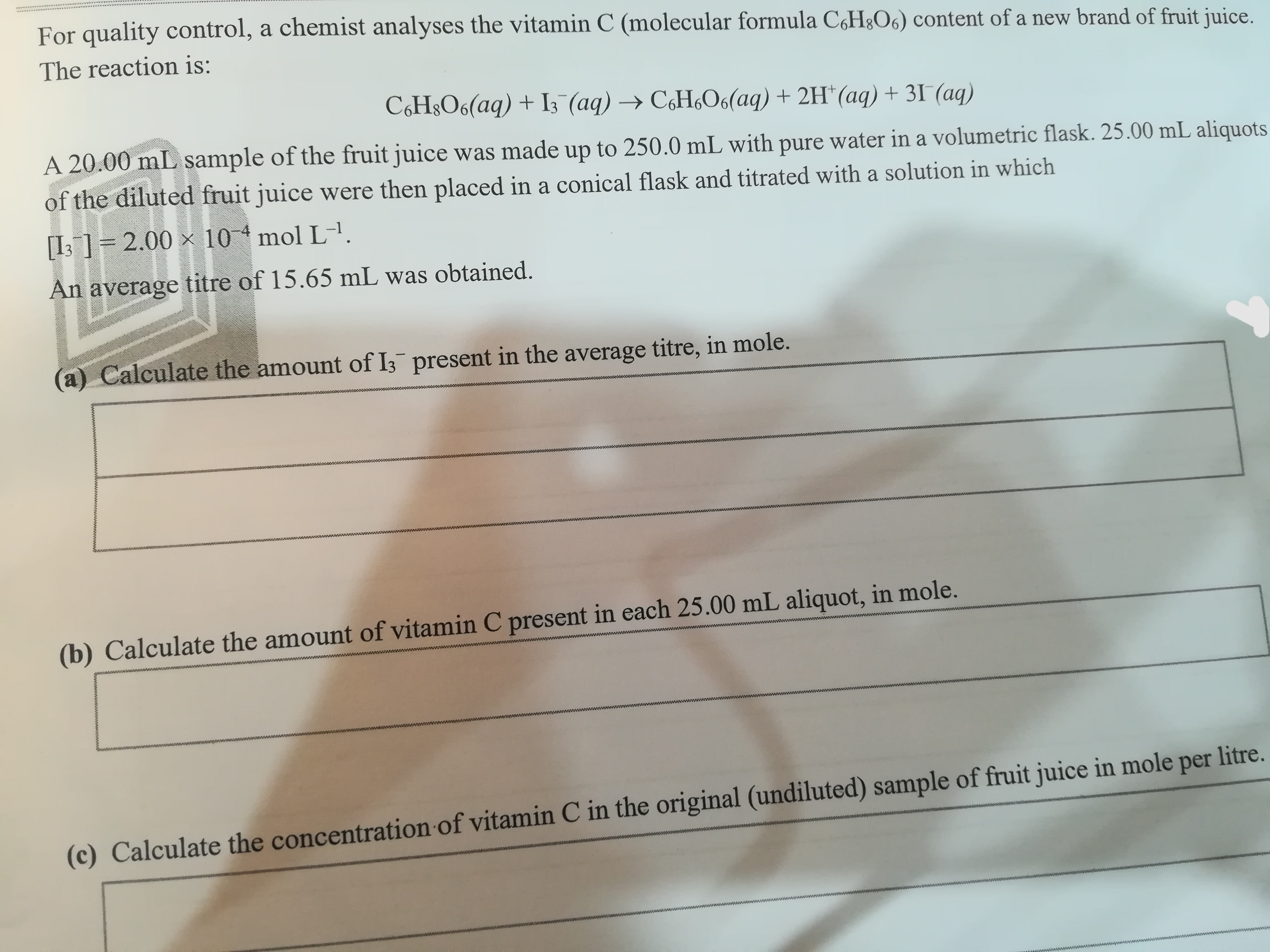# For quality control, a chemist analyses the vitamin C (molecular formula CH&O6) content of a new brand of fruit juice.The reaction is:C6H&O6(aq) + I3 (aq)CH6O6(aq) + 2H (aq) +31 (aq)A 20.00 mL sample of the fruit juice was made up to 250.0 mL with pure water in a volumetric flask. 25.00 mL aliquotsof the diluted fruit juice were then placed in a conical flask and titrated with a solution in which[I3] 2.00 x 104 mol L1An average titre of 15.65 mL was obtained.(a) Calculate the amount of I3 present in the average titre, in mole.(b) Calculate the amount of vitamin C present in each 25.00 mL aliquot, in mole.(c) Calculate the concentration of vitamin C in the original (undiluted) sample of fruit juice in mole per litre.

Question
4 views

Question in imagehelp_outlineImage TranscriptioncloseFor quality control, a chemist analyses the vitamin C (molecular formula CH&O6) content of a new brand of fruit juice. The reaction is: C6H&O6(aq) + I3 (aq) CH6O6(aq) + 2H (aq) +31 (aq) A 20.00 mL sample of the fruit juice was made up to 250.0 mL with pure water in a volumetric flask. 25.00 mL aliquots of the diluted fruit juice were then placed in a conical flask and titrated with a solution in which [I3] 2.00 x 104 mol L1 An average titre of 15.65 mL was obtained. (a) Calculate the amount of I3 present in the average titre, in mole. (b) Calculate the amount of vitamin C present in each 25.00 mL aliquot, in mole. (c) Calculate the concentration of vitamin C in the original (undiluted) sample of fruit juice in mole per litre. fullscreen
check_circle

Step 1

(a) The volume of the average titer is 15.65 mL or 0.01565 L. The number of moles of triiodide ion (n1) is calculated using equation (1) in which C1 is the concentration of triiodide ion solution and V1 is the volume of solution.

Step 2

(b) According to the reaction, one mole of vitamin C reacts with one mole of triiodide ion. The number of moles of vitamin C in a 25 mL solution is equal to the number of moles of triiodide present in a 15.65 mL triiodide solution. So, the amount of vitamin C (n2) in each 25 mL aliquot is 3.13×10-6 mol.

Step 3

(c) The concentration of vitamin C (C2) in 25 mL or 0.025 L solution is calculated using equation (2) in which n2...

### Want to see the full answer?

See Solution

#### Want to see this answer and more?

Solutions are written by subject experts who are available 24/7. Questions are typically answered within 1 hour.*

See Solution
*Response times may vary by subject and question.
Tagged in

### Solutions